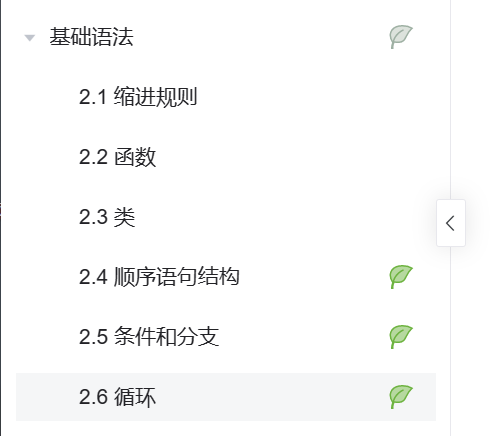# 2023（春）Python程序设计作业1： 基础语法之控制流程

## 一、完成技能树基础语法部分练习，如下：## 二、发表博客，内容为基础语法部分的学习笔记。

1.注释

#这是一行注释
print("Hello, World!")

2.变量

x = 5
y = "Hello, World!"

3.数据类型
Python支持多种数据类型，包括整数、浮点数、布尔值、字符串和列表等。

x = 5

x = 3.14

x = True
y = False

x = "Hello, World!"
y = 'Python is fun!'

x = [1, 2, 3, 4, 5]
y = ["apple", "banana", "cherry"]

4.数学运算

x = 5
y = 10
z = x + y
print(z) #输出15

x = 10
y = 5
z = x - y
print(z) #输出5

x = 2
y = 3
z = x * y
print(z) #输出6

x = 10
y = 2
z = x / y
print(z) #输出5.0

x = 10
y = 3
z = x % y
print(z) #输出1

5.字符串操作

x = "Hello"
y = "World"
z = x + " " + y
print(z) #输出Hello World

x = "Hello"
y = x * 3
print(y) #输出HelloHelloHello

x = "Hello, World!"
y = x[0:5]
print(y) #输出Hello

x = "Hello, World!"
y = x.find("World")
print(y) #输出7

6.列表操作

x = [1, 2, 3]
x.append(4)
print(x) #输出[1, 2, 3, 4]

x = [1, 2, 3]
x.remove(2)
print(x) #输出[1, 3]

x = [1, 2, 3]
x.insert(1, 4)
print(x) #输出[1, 4, 2, 3]

x = [1, 2, 3, 4, 5]
y = x[0:3]
print(y) #输出[1, 2, 3]

x = [1, 2, 3, 4, 5]
y = x.index(3)
print(y) #输出2

7.条件语句

if语句：
x = 10
if x > 5:
print("x是大于5的数")
if-else语句：
x = 10
if x > 5:
print("x是大于5的数")
else:
print("x是小于等于5的数")
if-elif-else语句：
x = 10
if x > 10:
print("x是大于10的数")
elif x > 5:
print("x是大于5小于等于10的数")
else:
print("x是小于等于5的数")

8.循环语句

while循环：
x = 0
while x < 5:
print(x)
x += 1
for循环：
x = [1, 2, 3, 4, 5]
for i in x:
print(i)

9.函数

def my_function():
print("Hello, World!")

my_function()

def my_function(name):
print("Hello, " + name + "!")

my_function("John")

def my_function(x, y):
return x + y

z = my_function(5, 10)
print(z) #输出15

...全文
18 回复 打赏 收藏 转发到动态 举报21南开大学《程序设计基础（上）》在线作业参考答案.docx
21南开大学《程序设计基础（上）》在线作业参考答案.docx2023Python程序设计作业5：Pandas基础技能及综合应用
【代码】2023Python程序设计作业5：Pandas基础技能及综合应用。2023Python程序设计作业6：基于unitest的Python测试
【代码】2023Python程序设计作业6：基于unitest的Python测试。21南开大学《程序设计基础（上）》在线作业-1参考答案.docx
21南开大学《程序设计基础（上）》在线作业-1参考答案.docx2023Python程序设计作业3： 函数及应用
if y == 0:else:x, y = 4，6二、Lambda函数n = 7三、递归函数if n == 1:return 1else:n = 5。

162发帖与我相关我的任务
python 高校 江苏省·南通市• 近7日
• 近30日
• 至今